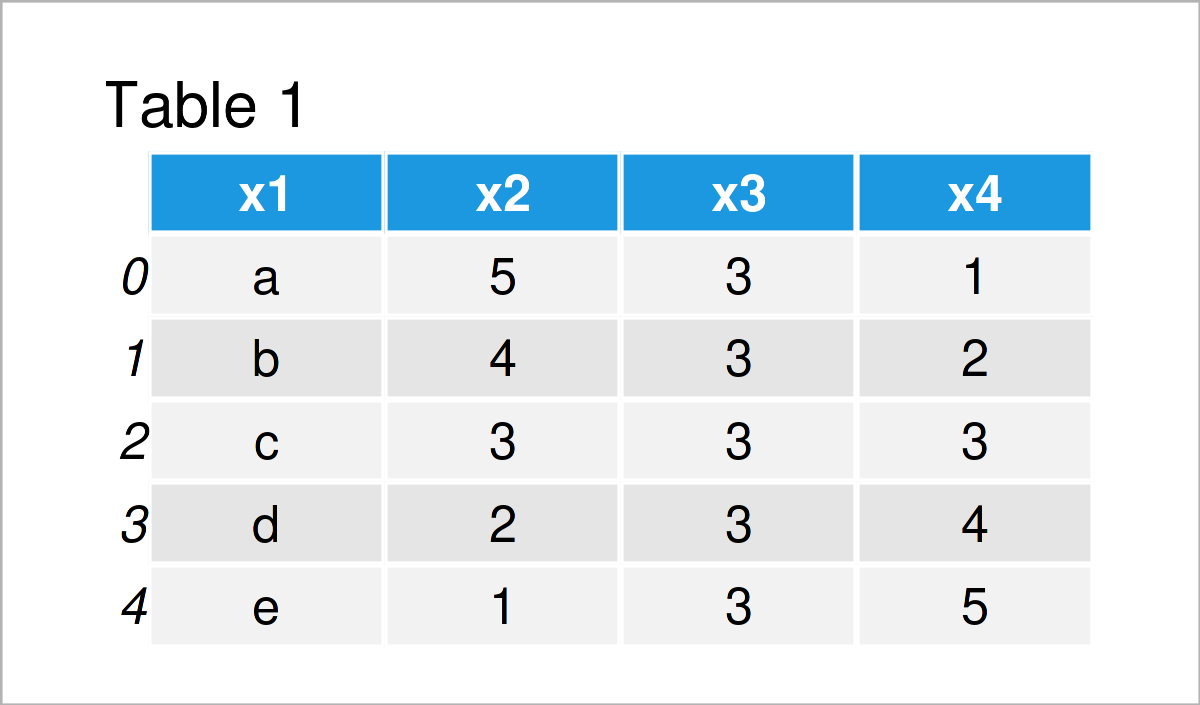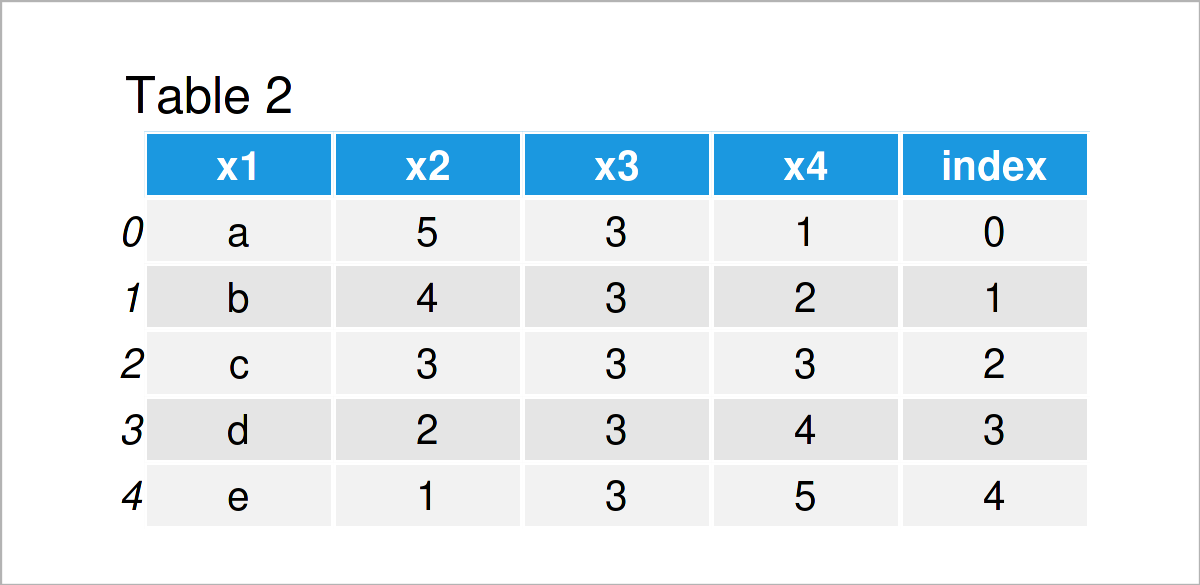# Convert Index to Column of pandas DataFrame in Python (Example)

In this Python programming tutorial you’ll learn how to add the index of a pandas DataFrame as a new variable.

The content looks as follows:

Let’s dive right into the example!

## Example Data & Add-On Libraries

First, we need to import the pandas library:

`import pandas as pd # Load pandas library`

We’ll use the following data as basement for this Python programming tutorial:

```data = pd.DataFrame({'x1':['a', 'b', 'c', 'd', 'e'], # Create example DataFrame 'x2':range(5, 0, - 1), 'x3':[3, 3, 3, 3, 3], 'x4':range(1, 6)}) print(data) # Print example DataFrame```Table 1 shows the structure of the exemplifying data – It has five rows and four columns.

## Example: Add Index as Column to pandas DataFrame

The following Python programming syntax illustrates how to convert the indices of a pandas DataFrame to a new column of this DataFrame.

For this, we can use the index attribute as shown in the following Python code:

```data_new = data.copy() # Duplicate DataFrame data_new['index'] = data_new.index # Convert index to variable print(data_new) # Print updated DataFrame```As shown in Table 2, the previous Python programming syntax has managed to create a new pandas DataFrame called data_new, which contains the index of the input data as an additional variable.

## Video & Further Resources

Please have a look at Corey Schafer’s video, in case you want to learn more about the handling of indices in Python. You can find the video below.

Please accept YouTube cookies to play this video. By accepting you will be accessing content from YouTube, a service provided by an external third party.If you accept this notice, your choice will be saved and the page will refresh.

In addition, you may want to have a look at the other articles on this website:

In this tutorial you have learned how to add the index of a pandas DataFrame as a new column in the Python programming language. Tell me about it in the comments section, in case you have further questions.

Subscribe to the Statistics Globe Newsletter